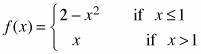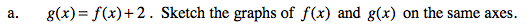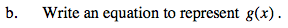### Home > PC > Chapter 2 > Lesson 2.3.2 > Problem2-90

2-90.
1. Let. 2-90 HW eTool (Desmos) Homework Help ✎

1. g(x) = f(x) + 2. Sketch the graphs of f(x) and g(x) on the same axes.

2. Write an equation to represent g(x).Shift the original function up 2 units.